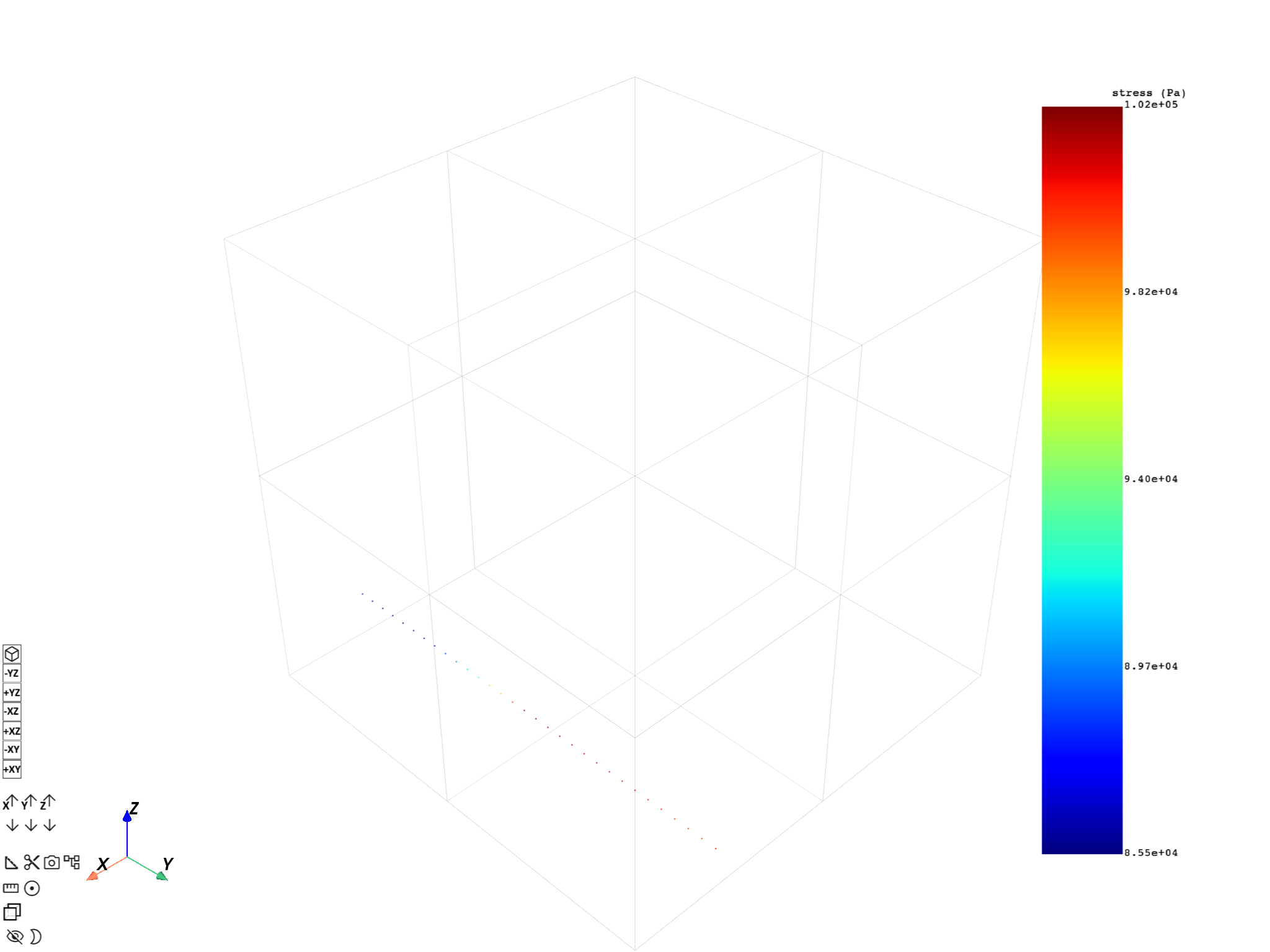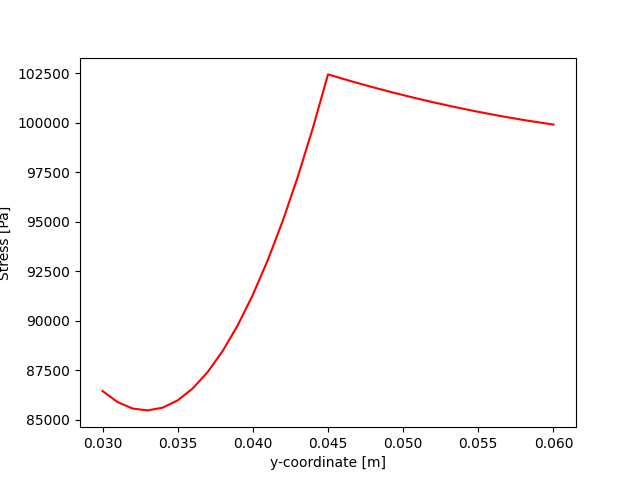# Plot results on a specific path#

This example shows how to get a result mapped over a specific path and how to plot it.

```import matplotlib.pyplot as plt

from ansys.dpf import core as dpf
from ansys.dpf.core import examples
from ansys.dpf.core import operators as ops
from ansys.dpf.core.plotter import DpfPlotter
```

# Plot path#

Use the `ansys.dpf.core.plotter.DpfPlotter` class to plot a mapped result over a defined path of coordinates.

```# Create the model and request its mesh and displacement data.
model = dpf.Model(examples.find_static_rst())
stress_fc = model.results.stress().eqv().eval()
```

Create a coordinates field to map on.

```coordinates = [[0.024, 0.03, 0.003]]
delta = 0.001
n_points = 51
for i in range(1, n_points):
coord_copy = coordinates.copy()
coord_copy = coord_copy + i * delta
coordinates.append(coord_copy)
field_coord = dpf.fields_factory.create_3d_vector_field(len(coordinates))
field_coord.data = coordinates
field_coord.scoping.ids = list(range(1, len(coordinates) + 1))
```

Compute the mapped data using the mapping operator.

```mapping_operator = ops.mapping.on_coordinates(
fields_container=stress_fc, coordinates=field_coord, create_support=True, mesh=mesh
)
fields_mapped = mapping_operator.outputs.fields_container()
```

Request the mapped field data and its mesh.

```field_m = fields_mapped
mesh_m = field_m.meshed_region
```

Create the plotter and add fields and meshes.

```pl = DpfPlotter()

# Plot the result.
pl.show_figure(show_axes=True)
```Plot the solution along the specified line. Note that since the line is only moving along the y-axis, the stresses are plotted with respect to the y coordinate.

```y_coords = [mesh_m.nodes.coordinates_field.data[i] for i in range(mesh_m.nodes.n_nodes)]
plt.plot(y_coords, field_m.data, "r")
plt.xlabel(f"y-coordinate [{mesh.unit}]")
plt.ylabel(f"Stress [{field_m.unit}]")
plt.show()
```Total running time of the script: ( 0 minutes 1.829 seconds)

Gallery generated by Sphinx-Gallery